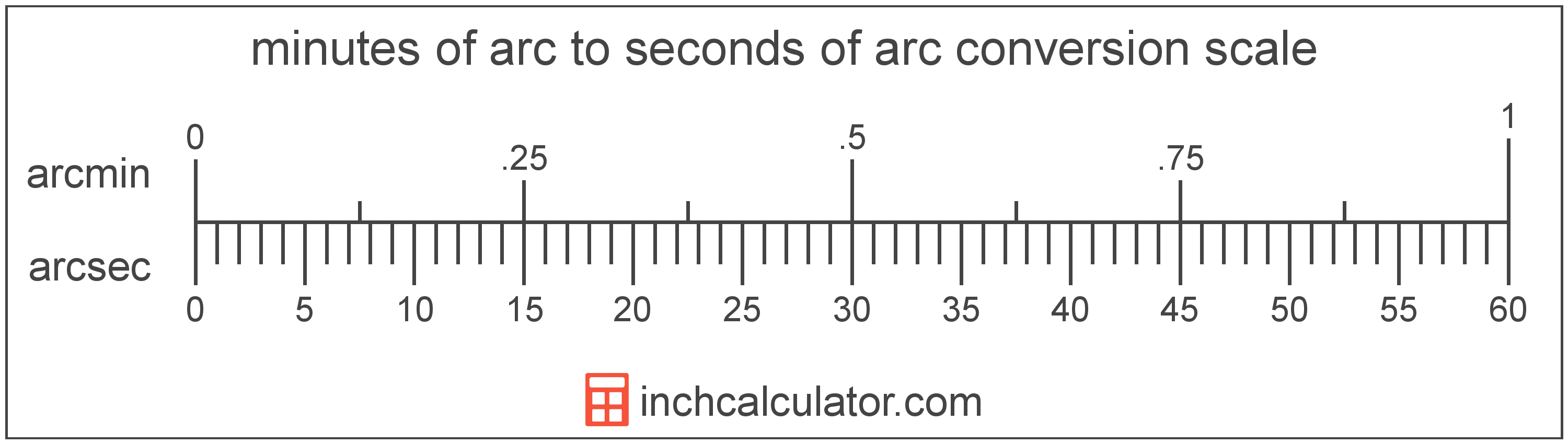# Minutes Of Arc to Seconds Of Arc Conversion

Enter the angle in minutes of arc below to get the value converted to seconds of arc.

Results in Seconds Of Arc:1 arcmin = 60 arcsec

## How to Convert Minutes Of Arc to Seconds Of ArcTo convert a minute of arc measurement to a second of arc measurement, multiply the angle by the conversion ratio.

Since one minute of arc is equal to 60 seconds of arc, you can use this simple formula to convert:

seconds of arc = minutes of arc × 60

The angle in seconds of arc is equal to the minutes of arc multiplied by 60.

For example, here's how to convert 5 minutes of arc to seconds of arc using the formula above.
5' = (5 × 60) = 300"

### How Many Seconds Of Arc are in a Minute Of Arc?

There are 60 seconds of arc in a minute of arc, which is why we use this value in the formula above.

1' = 60"

Minutes of arc and seconds of arc are both units used to measure angle. Keep reading to learn more about each unit of measure.

## Minutes Of Arc

The minute of arc is a unit of angle equal to 1/60th of one degree, or 1/21,600 of a circle. The minute of arc is also equal to π/10,800 radians.

A minute of arc is sometimes also referred to as an arc minute, arcminute, or minute arc. Minutes of arc can be abbreviated as arcmin, and are also sometimes abbreviated as MOA or amin. For example, 1 minute of arc can be written as 1 arcmin, 1 MOA, or 1 amin.

The minute of arc is most commonly represented using the prime (′), although the single-quote is commonly used. For instance, 1 minute of is most commonly expressed as 1′.

## Seconds Of Arc

The second of arc is a unit of angle equal to 1/60th of one minute of arc or 1/3,600 of one degree.

A second of arc is sometimes also referred to as an arc second or arcsecond. Seconds of arc can be abbreviated as arcsec, and are also sometimes abbreviated as asec. For example, 1 second of arc can be written as 1 arcsec or 1 asec.

The second of arc is most commonly expressed using a double prime (″), though a double quote is often used as well. For example, 1 second of arc is most often written as 1″.

## Minute Of Arc to Second Of Arc Conversion Table

Minute of arc measurements converted to seconds of arc
Minutes Of Arc Seconds Of Arc
1' 60"
2' 120"
3' 180"
4' 240"
5' 300"
6' 360"
7' 420"
8' 480"
9' 540"
10' 600"
11' 660"
12' 720"
13' 780"
14' 840"
15' 900"
16' 960"
17' 1,020"
18' 1,080"
19' 1,140"
20' 1,200"
21' 1,260"
22' 1,320"
23' 1,380"
24' 1,440"
25' 1,500"
26' 1,560"
27' 1,620"
28' 1,680"
29' 1,740"
30' 1,800"
31' 1,860"
32' 1,920"
33' 1,980"
34' 2,040"
35' 2,100"
36' 2,160"
37' 2,220"
38' 2,280"
39' 2,340"
40' 2,400"